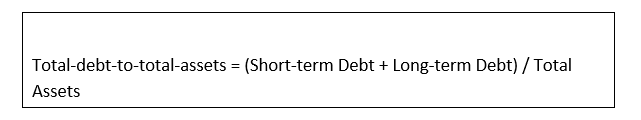# Total-Debt-to-Total-Assets ratio

• Updated on

What is the Total-Debt-to-Total-Assets Ratio?

Total-debt-to-total-assets ratio refers to the ratio can be described a leverage ratio that represents the amount of debt used to finance the assets of a company. It is commonly known as debt-to-asset ratio.

Summary
• Total-debt-to-total-assets ratio is the leverage ratio that represents the amount of debt used to finance the assets by a company.
• The higher total-debt-to-asset ratio indicates greater degree of leverage and financial risk.
• The ratio is used by creditors, analysts, and investors to measure the overall risk of a company.

Understanding Total-Debt-to-Total-Assets Ratio

The total-debt-to-asset ratio is the ratio used by creditors to estimate the total amount of debt of a company in order to know the repayment ability of a company whether additional advances and loan can be extended or not. In other words, we can say that investors and creditors use this ratio to ensure the solvency of a company. The ratio helps them to analyze or determine the ability of a company to meet their present and future obligations and capable of generating return on their investment.

The total-debt-to-total-assets ratio is used by the investors and creditors through evaluating the financial statement of a company such as balance sheet. Balance sheet includes all the debts of a company including short term and long term and all the assets of a company including tangible, intangible assets. Intangible assets of a company refer to those assets which cannot be seen and touched, no physical existence such as goodwill. The total-debt-to-total-assets ratio helps to determine how much debt a company uses to carry assets of a company by measuring leverage degree of a company.

The higher total-debt-to-asset ratio indicates the greater degree of leverage and financial risk. A company has to make its debt servicing payments in any circumstances; in case of breach of debt servicing payments which are not negotiated, company would face the risk of being enforced bankruptcy by its creditors. There are liabilities which can be negotiated to some extent such as long-term leases and accounts payable.What does the total-debt-to-total-assets ratio interprets?

Total-debt-to-total-assets ratio can be defined as a measure of the total amount of debt that is used to finance the assets of a company. Total-debt-to-total-asset is a leverage ratio that represents that how a company handles its assets. The ratio is used by creditors, analysts, and investors to measure the overall risk of a company.  More leveraged companies are considered more risky to invest and loan. More leveraged companies have to pay a large amount of its profit in interest payments and principle.

• If total-debt-to-total-assets ratio of a company equals to one, it represents that a company is highly leveraged and owns equal amount of liabilities as it assets. The company’s assets are equals to company’s liabilities.
• If total-debt-to-total-assets ratio of a company is more than one, it represents that a company’s liabilities are greater than its assets and extremely leveraged. In other words, a company owns more liabilities than its assets. Extremely leveraged companies involve high degree of risk to invest or to lend.
• If total-debt-to-total-assets ratio of a company is less than one, it represents that a company is least risky to invest and lend to. It shows that a company has more assets than its liabilities and in case of emergency a company can pay its current and future obligations by selling its assets.

Significance of the total-Debt-to-total-assets ratio

The total-debt-to-total-assets ratio is important for investors, analysts, and creditors as it shows the overall risk of company. The total-debt-to-total-assets ratio determines the risk by telling how much amount is owned by creditors in the relation of the amount of assets owned by the shareholders of the business. The ratio is used by the investors and the creditors to measure the debt capacity of a company; debt capacity of a company shows both debt servicing payments ability of a company and ability to generate amount from new debts.

Investors compare the total-Debt-to-total-assets ratio of different companies that belong to same industry to measure the ability of a company to raise cash from its new debts. The higher a company’s total-debt-to-total-assets ratio, the highly it is leveraged. Companies which have high leverage are said to be riskier as they have higher chances of missing debt payments.

From the creditors point of view, the total-debt-to-total-assets ratio is important to determine the total amount of debt a company already owned and whether a company is capable to repay its current debt. This will help creditor to take decision whether additional loans will be offered to the company.

What is the demerit of the total-debt-to-total-assets ratio?

There is only one shortcoming as per investors, analysts and creditors. The ratio does not give any sign of asset quality as it include all tangible and intangible assets together. The total-debt-to-total assets ratio is used to measures the total assets owned by a company that are financed by debt.

How is total-debt-to-total-assets ratio calculated?

The total-debt-to-total-assets ratio can be calculated by using the given formula:A company’s total-debt-to-total-assets ratio is calculated by dividing the total debt of a company with its total assets. Total debts include the short term and long-term debt and total assets include both tangible and intangible assets. For instance, XYZ has recorded total debt of £300,000 and total assets of £400,000 in the balance sheet.  The total-debt-to-total-assets ratio is calculated as

Total-debt-to-total-assets ratio = £300000 / £400000

= 0.75

##### Top ASX Listed Companies

Definition:
We use cookies to ensure that we give you the best experience on our website. If you continue to use this site we will assume that you are happy with it. OK